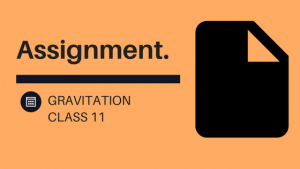Home » General » Gravitation assignment class 11

# Gravitation assignment class 11In this article find Gravitation assignment class 11. This is for your practice when you have completed the chapter and want to know where you stand in terms of the concepts. If you want the notes in this chapter please follow the link given below
gravitation class 11


Other links you might be interested in are

## (A) Conceptual problems on Gravitation

1. When an apple falls towards the earth, the earth moves up to meet the apple. Is this true? If yes, why is earth’s motion not noticeable?
2. Mention one point of difference between $G$ and $g$.
3. A satellite does not need any fuel to circle around Earth. Why?
4. How does weight of a body vary enroute from earth to moon? Would its mass change?
5. Is it possible to shield the body from gravitational effects? Give reason to support your answer.
6. A person in an artificial satellite of earth feels weightlessness. But a person on moon has weight though the moon is also a satellite of earth. Why?
7. Is it appropriate to describe the condition of weightlessness as condition of masslessness?
8. What velocity will you give to a donkey and what velocity would you give to a monkey do that both escape the gravitational field of the earth.
9. Where does a body weigh more :- at surface of earth or in a mine.
10. How is it that the moon is continuously circling around the earth and never falls towards the earth.

## (B) Definition and derivation type questions

1. Assuming the earth to be a sphere of uniform density , derive an expression for the density of the earth in terms of $G$ , $g$ , and its radius.
2. Discuss how acceleration due to gravity varies
(a) from point to point on earth’s surface(b) height above the earth’s surface(c) depth below the earth’s surface
3. (a) Define gravitational field intensity of gravitational field at a point. What are its units of intensity?
(b) Derive an expression for the gravitational field intensity due to a point mass M at a distance r from it. Also show that it is equal to free fall acceleration of mass at that point.
4. Derive an expression for the increase in potential potential energy of a body , when moved from point at a distance $r_1$ to a point at a distance $r_2$ , $(r_1 < r_2)$. Hence prove that increase in potential energy of a body of mass $m$ , when raised through a small distance $h$ above the surface of the earth is $mgh$.
5. What is escape velocity? Obtain the expression for the escape velocity on the earth.
6. What is a geostationary satellite? state the conditions , which are necessary , for a satellite to appear stationary.
7. What is a satellite? Obtain expression for(a) orbital velocity(b) period of revolution(c) height of the orbitabove the surface of earth.How do the expressions for orbital velocity and time period modify , when the orbit of the satellite is just above the surface of the earth.
8. What is weightlessness? Why does an astronaut feel weightless in an orbiting satellite?
9. State and explain the Kepler’s law of planetary motion.
10. Explain, how mass of sun can be found by studying the motion of the earth around it.

## (C) Gravitation extra questions

1. The time period of a satellite of earth is 5 hour. If the separation between earth and the satellite is increased to 4 times the previous value, then find the new time period. (Answer:- 40 hour)
2. Show that the Kepler’s third law of motion follows from the law of conservation of angular momentum.
3. The moon revolves around the earth and the earth moon system revolves around the sun. If the earth could be removed suddenly without disturbing the motion of the moon, what would be the subsequent path of the moon?
4. Among the known type of forces in nature, the gravitational force is the weakest. why then does it play a dominant role for the motion of bodies on the terrestrial , astronomical and cosmological scale?
5. What are the conditions under which a rocket fired from the earth becomes a satellite of the earth and orbits in a circle.
6. What will be the kinetic energy needed to project a body of mass $m$ from the earth’s surface (radius $R$) to infinity.
7. If $T$ is the time period of a satellite revolving just above the surface of a planet , whose average density is $\rho$ , show that $\rho T^2$ is a universal constant.
8. If you compare the gravitational force on the Earth due to the Sun to that due to the Moon you would find that the Sun’s pull is greater than the Moon’s pull. (You can check this yourself using the data available in the succeeding exercises). However, the tidal effect of the Moon’s pull is greater than the tidal effect of Sun. Why?
9. Suppose there existed a planet that went around the sun twice as fast as the earth. What would be its orbital size as compared to that of the earth?
10. Find the velocity of escape at the moon given that its radius is $1.7 \times 10^6 m$ and the value of $g$ at its surface is $1.63 ms^{-2}$. (Answer:- $2.354 \times 10^3 ms^{-1}$

## (D) Gravitation derivation class 11

Given below is the list of derivations you must remember and practice for gravitation class 11.

1. Derivation of 1st 2nd and 3rd law of Kepler s planetary motion
2. gravitational potential energy
3. variation of ‘g’ with altitude and depth
4. calculation of ‘g’
5. escape velocity of a satelite
6. orbital velocity of a satelite
7. Height of geostationary satellite
Subscribe
Notify of

This site uses Akismet to reduce spam. Learn how your comment data is processed.